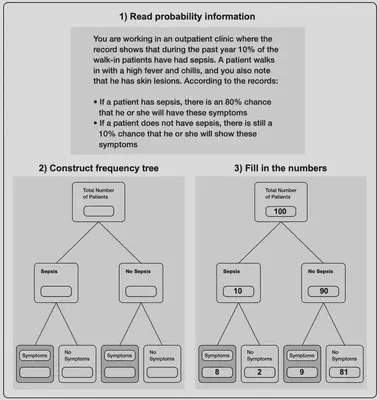# Teaching how to construct natural frequency trees out of probability information

### What is the boost?

Knowing how to construct natural frequency trees out of probability information helps people successfully make Bayesian inferences (e.g., to understand what a positive test result really means).Copyright © 2001 by APA. Adapted with permission. “Teaching Bayesian reasoning in less than two hours,” by P. Sedlmeier and G. Gigerenzer, 2001, Journal of Experimental Psychology: General, 130(3). https://doi.org/10.1037/0096-3445.130.3.380

The boost above involves four steps:

1. Read the problem description represented in probabilities.
2. Draw a natural frequency tree. The root node (at the top) represents the total number of cases and is then broken down into four subclasses.
3. Fill in the numbers. For convenience, set the root node to 100 patients. Insert the base-rate frequency in the “sepsis” node by calculating 10% of 100 patients (= 10). Fill in the “no sepsis” node by subtracting the patients with sepsis from the total number of patients (100 - 10 = 90). Divide the 10 patients in the sepsis node into 8 showing the symptoms (80%) and 2 not showing the symptoms (the remaining 20%). Divide the 90 patients in the “no sepsis” node into 9 showing the symptoms (90%) and 81 not showing the symptoms (the remaining 10%).
4. Calculate the posterior probability. With all numbers filled in, the probability of sepsis given the presence of symptoms can be easily calculated: \$8/(8 + 9) = .47\$ or 47%.

### Which challenges does the boost tackle?

Risks that are communicated using opaque statistical representations based on conditional probabilities can be difficult to grasp (see the natural frequencies section).

### Which competences does the boost foster?

The ability to translate probability information into a natural frequency tree, then to apply Bayes’s rule to calculate a posterior probability.

### What is the evidence behind it?

Sedlmeier and Gigerenzer (2001) offered training in natural frequency trees. They compared it with training in conditional probability trees and rule-based training in Bayes’s rule. Although the immediate effect was strong for all training programs, only the training in natural frequency trees showed no decay over time: The immediate training effect of a median of 93% Bayesian solutions, compared to a median of 14% at baseline, remained stable over a period of 15 weeks.

### Key reference

Sedlmeier, P., & Gigerenzer, G. (2001). Teaching Bayesian reasoning in less than two hours. Journal of Experimental Psychology: General, 130(3), 380–400. https://doi.org/10.1037/0096-3445.130.3.380